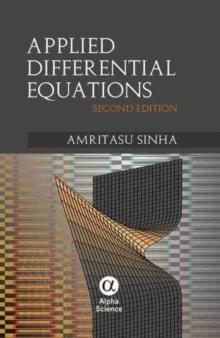Supporting your high street Find out how »
• My Account# Applied Differential Equations Hardback

#### Description

Applied Differential Equations discusses the Legendre and Bessel Differential equations and its solutions.

Various properties of Legendre Polynomials as well as Legendre function and Bessel functions in part one.

The second order Partial Differential equation of three types is studied and the technique to solve with the separation of variables technique called Fourier's Method have been discussed in the second part.

In the Appendix some applications of the Heat Equation are discussed to Model the Environment.

NEW TO THE SECOND EDITION: Chapter on Matlab Solution to ODE, PDE and SDE as an appendix In the Appendix some applications of the Heat Equation are discussed to Model the Environment.

#### Information

• Format: Hardback
• Pages: 224 pages
• Publisher: Alpha Science International Ltd
• Publication Date:
• Category: Differential calculus & equations
• ISBN: 9781842658055

#### Other Formats

£54.95

£45.35